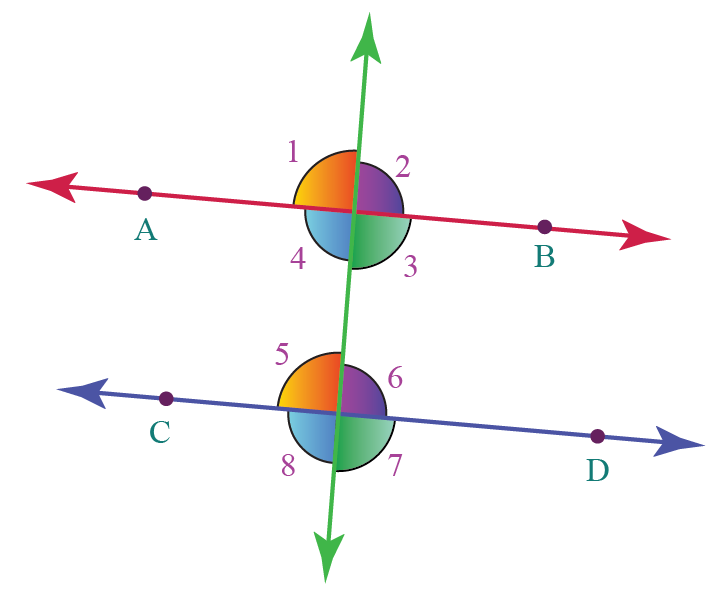# Alternate Angles

Alternate Angles
Go back to  'Angles'

You must have studied or heard about various types of angles so far formed by a transversal falling on two lines. One such type of angle is alternate angles.

In the simulation below, tick the box to visualize the alternate angles. You can drag the point of intersection to change the values of angles.

In this mini-lesson, we will explore more about angles by learning about alternate angles including its definition, types of alternate angles, theorems on alternate angles with the help of interesting simulation, some solved examples and a few interactive questions for you to test your understanding.

## Lesson Plan

 1 What Are Alternate Angles? 2 Important Notes on Alternate Angles 3 Solved Examples on Alternate Angles 4 Challenging Questions on Alternate Angles 5 Interactive Questions on Alternate Angles

## What Are Alternate Angles?

When two straight lines are cut by a transversal, then the angles formed on the opposite side of the transversal with respect to both the lines are called alternate angles.

So, alternate angles are those angles that:

• have different vertices
• lie on the alternate sides of the transversalThe pairs of alternate angles in the above figure are:

$$\angle 3$$ and $$\angle 5$$

$$\angle 4$$ and $$\angle 6$$

$$\angle 1$$ and $$\angle 7$$

$$\angle 2$$ and $$\angle 8$$

## What Are the Types of Alternate Angles?

There are two types of alternate angles- alternate interior angles and alternate exterior angles.

Alternate angles that lie in the interior region of both the lines are called alternate interior angles.

Alternate angles that lie in the exterior region of both the lines are called alternate exterior angles

### Example

In the above image, two pairs of alternate interior angles are $$\angle 3$$ and $$\angle 5$$ and $$\angle 4$$ and $$\angle 6$$.

two pairs of alternate exterior angles are $$\angle 2$$ and $$\angle 8$$ and $$\angle 1$$ and $$\angle 7$$.

More Important Topics
Numbers
Algebra
Geometry
Measurement
Money
Data
Trigonometry
Calculus